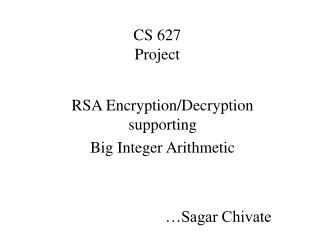DownloadDownload PresentationCS 627 Project

# CS 627 Project

Download Presentation## CS 627 Project

- - - - - - - - - - - - - - - - - - - - - - - - - - - E N D - - - - - - - - - - - - - - - - - - - - - - - - - - -
##### Presentation Transcript

1. CS 627Project RSA Encryption/Decryption supporting Big Integer Arithmetic …Sagar Chivate

2. Motivation • Curiosity to know how the big integer calculations are performed in real world • Why RSA RSA allowed me to develop my own encryption and decryption algorithms, instead of using some already existing ones

3. Functionality • Encryption Take file to be encrypted from user Take encryption key, n, output file from user Encrypt file and store encrypted file • Decryption Take file to be decrypted from user Take decryption key, n, output file from user Decrypt file and store decrypted file

4. Other Functionality • Find d from e Takes d and output file from user Uses Euclid’s algorithm Calculates e and stores it • Find prime Takes number of bits, output file from user Calculates and stores prime of specified bit order • Find N Calculates and stores N taking P and Q as input

5. VB (exe) VC++ (dll) VC++ (lib) Encrypt / Decrypt User Inter-face Big Integer Calc. bigint.lib encryptdecrypt.dll RSA.exe System Architecture

6. Development: Step1 Develop bigint.lib • Go to http://www.shoup.net/ntl/ • Download NTL(library to do number theory) • Create a VC++ project: win32statically linked library • Add all (96) files (.cpp and .h)in downloaded src folder to the project • Set category: Preprocessor, specify include path in directories • Build the project. It should successfully (with 49 warnings) create .lib file (size around 10MB)

7. About NTL • Created by Victor Shoup (IBM) • NTL is a high-performance, portable C++ library providing data structures and algorithms for manipulating signed, arbitrary length integers, and for vectors, matrices, and polynomials over the integers and over finite fields • For big integers it supports: All basic mathematical operations (+, -,/,*,exp,mod,sqrt) Data type conversions Find GCD,primes,primality checking,next prime Bitwise operations Random number generation etc…

8. Implementation details of NTL • ZZ is big integer data type implemented in NTL. It is actually a structure, which as a whole holds the big integer • Most of the normal arithmetic operators are overloaded for ZZ data type • All the calculation are performed at the bit level • The maximum integer this can handle, is of the order of 22^15 bits integer. This is because the number of bits is handled as “long” (15 digit precision) everywhere, which puts the limit on the maximum order of the integer

9. Development: Step2 Develop encryptdecrypt.dll • Create a VC++ project: win32dynamic-link library • Implement methods for: Encryption, Decryption, calculate d from e, find prime, calculate n in a .cpp file • Use ZZ data type and inbuilt functions in bigint.lib to handle big integer arithmetic. Refer ZZ.h for prototypes of all supported functions • Throw the errors (if any) to calling RSA.exe • Create a .def (definition) file to specify dll entry points Build the project to create dll file

10. Development: Step3 Develop RSA.exe • Create standard exe VB project • Design and implement user interface • Responsible for all user input/output and file handling • Write wrapper functions for all the implemented methods in encryptdecrypt.dll • Use encryptdecrypt.dll methods to achieve functionality • Handle errors and prompt user with the perceived error • Create executable (RSA.exe) file

11. Testing • Generate two huge primes, p and q (of the order of 2048 bits) • Select e (small to speed up encryption) • Find d from e • Encrypt any file • Decrypt encrypted file • Compare original file with the decrypted one. Ideally(!), they should match

12. Links • ARIBAS: It has own Pascal like scripting language. You can write your own functions. But its functionality is limited to handle mathematical operations. • LiDIA: A C++ Library For Computational Number Theory. The LiDIA Group at the Darmstadt University of Technology developed LiDIA. • Bit::Vector: It is an efficient C library which allows you to handle "big integer arithmetic" There are also Perl wrapper modules developed for Bit::Vector • BeeCrypt:Java's BigInteger library • MegaNumberS: It is prime number and very large integer R&D web site. • NTL: Library to do number theory